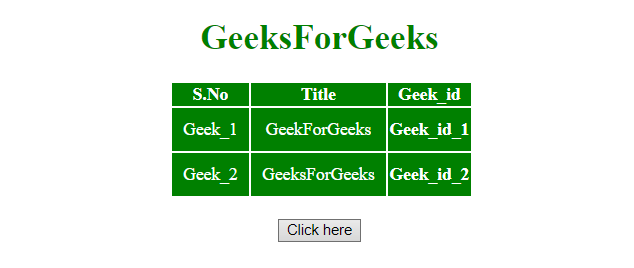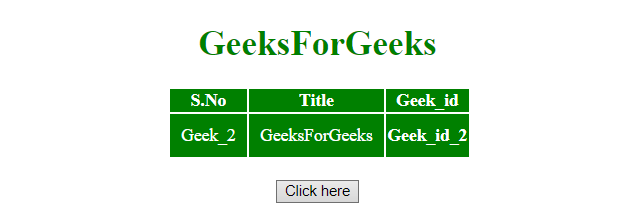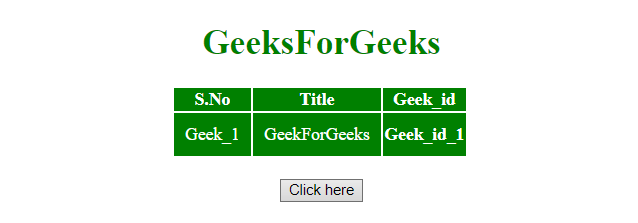# How to remove table row from table using jQuery ?

The jQuery remove() method is used to remove a row from HTML table.
jQuery remove() Method: This method removes the selected elements alongwith text and child nodes. This method also removes data and events of the selected elements.

Syntax:

`\$(selector).remove(selector)`

Parameters: It accepts single parameter selector which is optional. It specifies one or more elements to be removed. If multiple elements are to be removed, separate them with a comma (,) operator.

Example 1: This example first selects the row by id value and then removes it by using remove() method.

 `  ` `<``html``>  ` `    ``<``head``>  ` `     `  `        ``<``title``>  ` `            ``How to remove a table row ` `            ``from table ` `        ``  ` `         `  `        ``<``script` `src` `=  ` `"https://ajax.googleapis.com/ajax/libs/jquery/3.4.0/jquery.min.js"``> ` `        `` ` ` `  `        ``<``style``> ` `            ``#myCol { ` `                ``background:green; ` `            ``} ` `            ``table { ` `                ``color:white; ` `            ``} ` `            ``#Geek_p { ` `                ``color:green; ` `                ``font-size:30px; ` `            ``} ` `            ``td { ` `                ``padding:10px; ` `            ``} ` `        `` ` `    ``  ` `     `  `    ``<``body``> ` `        ``<``center``>  ` `            ``<``h1` `style` `= ``"color:green;"` `>  ` `                ``GeeksForGeeks  ` `            ``  ` `                 `  `            ``<``table``> ` `                ``<``colgroup``> ` `                    ``<``col` `id``=``"myCol"` `                        ``span``=``"2"``> ` `                    ``<``col` `style``=``"background-color:green"``> ` `                `` ` `                 `  `                ``<``tr``> ` `                    ``<``th``>S.No ` `                    ``<``th``>Title ` `                    ``<``th``>Geek_id ` `                `` ` `                ``<``tr` `id` `= ``"row1"``> ` `                    ``<``td``>Geek_1 ` `                    ``<``td``>GeekForGeeks ` `                    ``<``th``>Geek_id_1 ` `                `` ` `                ``<``tr``> ` `                    ``<``td``>Geek_2 ` `                    ``<``td``>GeeksForGeeks ` `                    ``<``th``>Geek_id_2 ` `                `` ` `            `` ` `            ``<``br``> ` `             `  `            ``<``button` `onclick` `= ``"Geeks()"``>  ` `                ``Click here ` `            ``  ` `             `  `            ``` `            ``<``script``>  ` `                ``function Geeks() { ` `                        ``\$("#row1").remove(); ` `                ``} ` `            `` ` `        `` ` `    ``  ` `                     `

Output:

• Before clicking on the button:• After clicking on the button:Example 2: This example first select the last <tr> element of its parent and removes it by using remove() method.

 `  ` `<``html``>  ` `    ``<``head``>  ` `        ``<``title``>  ` `            ``How to remove table row ` `            ``from table ` `        ``  ` `         `  `        ``<``script` `src` `=  ` `"https://ajax.googleapis.com/ajax/libs/jquery/3.4.0/jquery.min.js"``> ` `        `` ` `     `  `        ``<``style``> ` `            ``#myCol { ` `                ``background:green; ` `            ``} ` `            ``table { ` `                ``color:white; ` `            ``} ` `            ``#Geek_p { ` `                ``color:green; ` `                ``font-size:30px; ` `            ``} ` `            ``td { ` `                ``padding:10px; ` `            ``} ` `        `` ` `    ``  ` `     `  `    ``<``body``> ` `        ``<``center``>  ` `            ``<``h1` `style` `= ``"color:green;"` `>  ` `                ``GeeksForGeeks  ` `            ``  ` `                 `  `            ``<``table``> ` `                ``<``colgroup``> ` `                    ``<``col` `id``=``"myCol"` `                        ``span``=``"2"``> ` `                    ``<``col` `style``=``"background-color:green"``> ` `                `` ` `                 `  `                ``<``tr``> ` `                    ``<``th``>S.No ` `                    ``<``th``>Title ` `                    ``<``th``>Geek_id ` `                `` ` `                ``<``tr` `id` `= ``"row1"``> ` `                    ``<``td``>Geek_1 ` `                    ``<``td``>GeekForGeeks ` `                    ``<``th``>Geek_id_1 ` `                `` ` `                ``<``tr``> ` `                    ``<``td``>Geek_2 ` `                    ``<``td``>GeeksForGeeks ` `                    ``<``th``>Geek_id_2 ` `                `` ` `            `` ` `            ``<``br``> ` `             `  `            ``<``button` `onclick` `= ``"Geeks()"``>  ` `                ``Click here ` `            ``  ` `             `  `            ``<``script``>  ` `                ``function Geeks() { ` `                    ``\$('tr:last-child').remove(); ` `                ``} ` `            ``  ` `        `` ` `    ``  ` `                     `

Output:

• Before clicking on the button:• After clicking on the button:My Personal Notes arrow_drop_upCheck out this Author's contributed articles.

If you like GeeksforGeeks and would like to contribute, you can also write an article using contribute.geeksforgeeks.org or mail your article to contribute@geeksforgeeks.org. See your article appearing on the GeeksforGeeks main page and help other Geeks.

Please Improve this article if you find anything incorrect by clicking on the "Improve Article" button below.